# KSEEB Solutions for Class 7 Maths Chapter 5 Lines and Angles Ex 5.1

Students can Download Chapter 5 Lines and Angles Ex 5.1, Question and Answers, Notes Pdf, KSEEB Solutions for Class 7 Maths, Karnataka State Board Solutions help you to revise complete Syllabus and score more marks in your examinations.

## Karnataka State Syllabus Class 7 Maths Chapter 5 Lines and Angles Ex 5.1

Question 1.
Find the complement of each of the following angles :
i) The complement of 70°
Solution: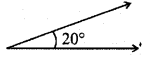The complement of the angle 20° = 90° – 20° = 70°

ii)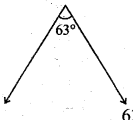The complement of the angle 63° = 90° – 63° = 27°

iii)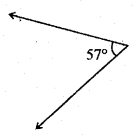The complement of the angle 57° = 90° – 57° = 33°Question 2.
Find the supplement of each of the following angles :
Solution:
i)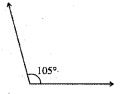The supplement of the angle 105°= 180° – 105° = 75°

ii)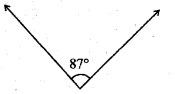The supplement of the angle 87°= 180° – 87° = 93°

iii) <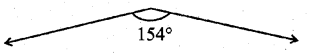The supplement of the angle 154°= 180° – 154° = 26°Question 3.
Identify which of the following pairs of angles are complementary and which are supplementary.
i) 65°, 115°
65° + 115° = 180°
∴ This pair is supplementary angles.

ii) 63°, 27°
63°+ 27° = 90°
∴ This pair is complementary angles.

iii) 112°, 68°
112°+68°= 1800
∴ This pair is supplementary angles.

iv) 130°, 50°
130° + 50° = 180°
∴ This pair is supplementary angles.

v) 45°, 45°
45° + 45° = 90°
∴ This pair is complementary angles.

vi) 80°, 10°
80°+ 10° = 90°
This pair is complementary angles.

Question 4.
Find the angle which is equal to its complement.
Solution:
Let one of the complement angle be x°
Its complement be = 90° – x
∴ According to the question
x° = 90° – x°
x° + x° = 90°
2x° = 90°
x = 90/2 = 45°Question 5.
Find the angle which is equal to its supplement.
Solution:
Let one of supplement be x°
Another supplement be = 180° – x°
According to the question = x° = 180° – x°
x° + x° = 180°
2x° = 180°
x° = 180/2 = 90°

Question 6.
In the given figure, ∠1 and ∠2 are supplementary angles.
Solution:
If ∠1 is decreased, what changes should take place in ∠2 so that both the angles still remain supplementary?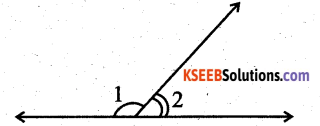If ∠1 is decreased the ∠2 will be increased.

Question 7.
Can two angles be supplementary if both of them are :
i) acute?
No, two acute angles cannot be supplementary. [∵ acute angles is ∠90°]

ii) obtuse?
No, two obtuse angles cannot be supplementary. [∵ obtuse angles is ∠90°].

iii) right?
Yes, Two right angles always supplementary. [∵ right angles is = 90°].

Question 8.
An angle is greater than 45°. Is its complementary angle greater than 45° or equal to 45° or less than 45°?
Solution:
If an angle is greater than 45° then its complement should be less than 45°.Question 9.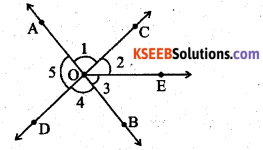i) Is ∠1 adjacent to ∠2?
Yes, ∠1 is adjacent to ∠2
ii) Is ∠AOC adjacent to ∠AOE?
No, ∠AOC is not adjacent to ∠AOE.
iii) Do ∠COE and ∠EOD form a linear pair?
Yes, ∠COE and ∠EOD are linear pairs.
iv) are ∠BOD and ∠DO A supplementary?
Yes, ∠BOD and ∠DOA are supplementary.
v) Is ∠1 vertically opposite to ∠4?
Yes, ∠1 is vertically opposite to ∠4.
vi) What is the vertically opposite angle of ∠5?
The vertically opposite angle of ∠5 is ∠2 + ∠3 ie., ∠COB.
∠COE + ∠EOB = ∠COBQuestion 10.
Indicate which pairs of angles are :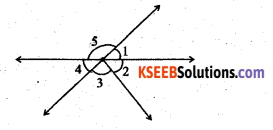i) Vertically opposite angles.
Vertically opposite angles are
∠1 and ∠4
∠5 and ∠2 + ∠3
ii) Linear pairs
Linear pairs
∠5 and ∠1
∠4 and ∠5

Question 11.
In the following figure, is ∠1 adjacent to ∠2 ? Give reasons.
Solution:∠1 is not adjacent to ∠2 because their vertex is not common.

Question 12.
Find the values of the angles x, y, and z in each of the following :
Solution:
Given < = 55°∠x = 55° (∵ vertically opposite angles)
∠y = 180° – 55° = 125° (∵ linear pair)
∠z = ∠y = 125°(∵ vertically opposite angles)

ii)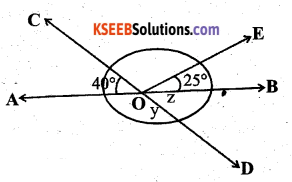Given
∠AOC = 40°
∠EOB = 25° .
AOB is a straight angIe (∵ AOB is st line)
∠AOB = ∠AOC + ∠EOB
180° = 40° + ∠COE + 25°
180° = 65°+ ∠COE
∴ ∠COE = 180° – 65° = 115°
∠y + ∠z = 180°
∠z = 40° (∵ vertically opposite angles)
∴ y = 180° – 40° = 140°Question 13.
Fill in the blanks :

1. If two angles are complementary, then the sum of their measures is 90°
2. If two angles are supplementary, then the sum of their measures is 180°
3. Two angles forming a linear pair are supplementary
4. If two adjacent angles are supplementary, they form a linear pair
5. If two lines intersect at a point, then the vertically opposite angles are always equal
6. If two lines intersect at a point, and if one pair of vertically opposite angles are acute angles, then the other pair of vertically opposite angles are obtuse angles.

Question 14.
In the adjoining figure, name the following pairs of angles.Solution:
i) Obtuse vertically opposite angles
∠AOD and ∠BOC